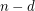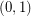Importance: High ✭✭✭
 Author(s): Hirsch, Warren M.
 Subject: Geometry » Polytopes
 Keywords: diameter polytope
 Posted by: Robert Samal on: May 11th, 2007
 Solved by: Francisco Santos
Conjecture   Letbe a convex-polytope withfacets. Then the diameter of the graph of the polytopeis at most.

(Originally appeared in [D], this discussion appears as [M].)

Many special cases of this conjecture have been verified, and in particular, the case of-polytopes was settled by Naddef by an elegant proof in [N], and further generalized in [MT]. However, in the general case, the Hirsch conjecture is known only for. It is even open if there is a polynomial upper bound on the diameter. Kalai and Kleitman [KK] found a quasi-polynomial bound by proving that the diameter is bounded by.

The special case of Hirsch Conjecture, the-step conjecture, asserts that the diameter is equal toif the polytope has preciselyfacets. The-step conjecture has been verified for, and similarly as the Hirsch Conjecture, it is completely open for higher dimensions. This has been the situation for the past 50 years, and there is no evidence for essential progress.

## Bibliography

*[D] G. B. Dantzig: Linear Programming and Extensions, Princeton University Press (1963).

[KK] G. Kalai and D. J. Kleitman, A quasi-polynomial bound for the diameter of graphs of polyhedra, Bull. Amer. Math. Soc. (N.S.) 26 (1992), 315-316.

[MT] T. Matsui and S. Tamura, Adjacency on combinatorial polyhedra, Discrete Appl. Math. 56 (1995) 311-321.

[N] D. Naddef, The Hirsch conjecture is true for (0,1)-polytopes, Math. Progr. (Ser. B) 45 (1989) 109-110.

[M] B. Mohar, Problem of the Month

* indicates original appearance(s) of problem.